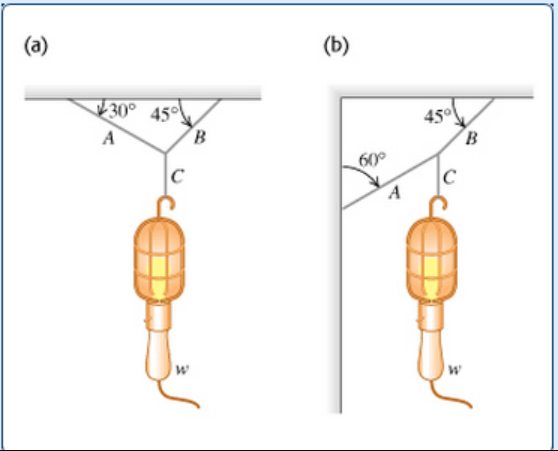# Problem: Find the tension in each cord in the figure if the weight of the suspended object is w.1) Find the tension of the cord A in the figure (a).2) Find the tension of the cord B in the figure (a).3) Find the tension of the cord C in the figure (a).4) Find the tension of the cord A in the figure (b).5) Find the tension of the cord B in the figure (b).6) Find the tension of the cord C in the figure (b).

###### FREE Expert Solution

For system a

1)

Tension in the cord:

$\overline{){{\mathbf{T}}}_{{\mathbf{c}}}{\mathbf{=}}{\mathbit{w}}}$

Tc = TA sin (30°) + TB sin (45°)

TA sin (30°) + TB sin (45°) = w

TA cos (30°) - TB cos (45°) = 0

99% (85 ratings)###### Problem DetailsFind the tension in each cord in the figure if the weight of the suspended object is w.

1) Find the tension of the cord A in the figure (a).

2) Find the tension of the cord B in the figure (a).

3) Find the tension of the cord C in the figure (a).

4) Find the tension of the cord A in the figure (b).

5) Find the tension of the cord B in the figure (b).

6) Find the tension of the cord C in the figure (b).